# KSEEB SSLC Class 10 Maths Solutions Chapter 11 Introduction to Trigonometry Ex 11.2

In this chapter, we provide KSEEB SSLC Class 10 Maths Solutions Solutions Chapter 11 Introduction to Trigonometry Ex 11.2 for English medium students, Which will very helpful for every student in their exams. Students can download the latest KSEEB SSLC Class 10 Maths Solutions Solutions Chapter 11 Introduction to Trigonometry Ex 11.2 pdf, free KSEEB SSLC Class 10 Maths Solutions Solutions Chapter 11 Introduction to Trigonometry Ex 11.2 pdf download. Now you will get step by step solution to each question.

### Karnataka State Syllabus Class 10 Maths SolutionsChapter 11 Introduction to Trigonometry Ex 11.2

Trigonometry Exercise 11.2 Solutions Question 1.
Evaluate the following :
i) sin 60° cos 30° + sin 30° cos 60°
ii) 2 tan2 45° + cos2 30° – sin2 60°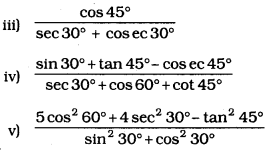Solution:
i) sin 60° cos 30° + sin 30° cos 60°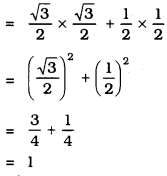ii) 2 tan2 45° + cos2 30° – sin260°
= 2(tan 45°)2 + (cos 30°)2 – (sin 60°)2
= 2 (1)2 + (3√2)2−(3√2)2
= 2 × 1
= 2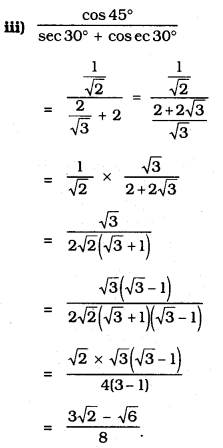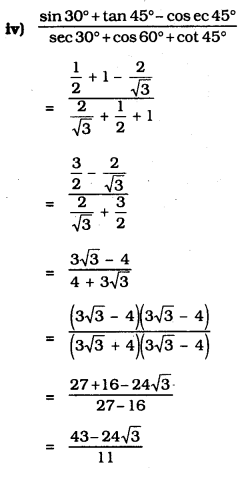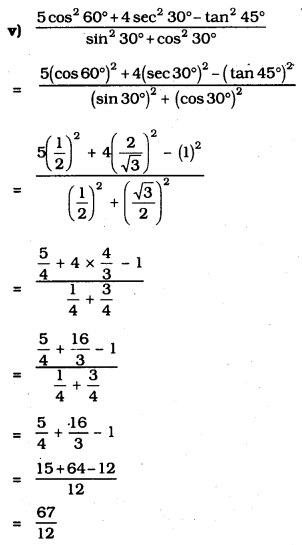Exercise 11.2 Class 10 Trigonometry Question 2.
Choose the correct option and justify your choice :
i) 2tan30∘1+tan230∘ =
A) sin 60°
B) cos 60°
C) tan 60°
D) sin 30°
Solution:
A) sin 60°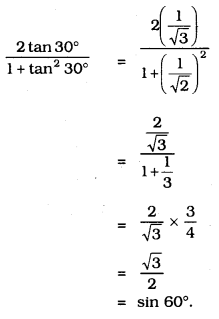ii) 1−tan245∘1+tan245∘ =
A) tan 90°
B) 1
C) sin 45°
D) 0
Solution:
D) 0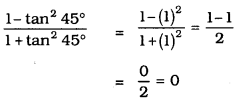iii) sin 2A = 2 sin A is true when A ;
A) 0°
B) 30°
C) 45°
D) 60°
Solution:
A) 0°
LHS = sin 2A = sin 0° = 0
RHS = 2sin A = 2.sin0° = 0

iv) 2tan30∘1−tan230∘ =
A) cos 60°
B) sin 60°
C) tan 60°
D) sin 30°
Solution:
C) tan 60°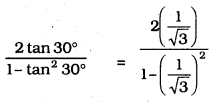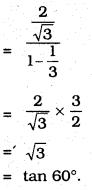Introduction To Trigonometry Exercise 11.2 Question 3.
If tan (A + B) =3–√ and tan (A – B) = 13√ 0° < A + B ≤ 90°; A > B. find A and B.
Solution:
tan (A + B) = 3–√
tan (A + B) = tan 60°
A + B = 60°
tan (A – B) = 13√ = tan 30°
tan(A – B) = tan 30°
A – B = 30° → (2)
A + B + A – B = 60 + 30
2A = 90
A = 902 = 45°
A = 45°
Put A = 45° in eqn (1)
A + B = 60
B = 60 – A= 60 – 45°
B = 15°.

Introduction To Trigonometry Class 10 Exercise 11.2 Question 4.
i) sin (A + B) = sin A + sin B
Solution:
False
Take A = 45° and B = 45°
LHS: sin (45° + 45°) = sin 90° = 1
RHS: sin 45 + sin 45 = 12√+12√=22√
LHS ≠ RHS.

ii) The value of sin θ increases as θ increases.
Solution:
True.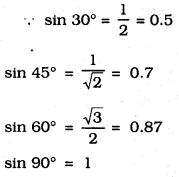∴ The value of sin θ increases as θ increases.

iii) The value of cos θ increases as θ increases.
Solution:
False.
cos 0° = 1 cos 30° = 3√2 =0.87
cos 45° = 12√ = 0.7 cos 60° = 12= = 0.5
cos 90° = 1
∴ The value of cos 0 increases as 0 increases – the statement is False.

iv) sin θ = cos θ for all values of θ.
Solution:
False.
sin 30° = 12 cos 30° = 3√2
sin 30° ≠ cos 30°
But sin 45° = cos 45° = 12√

v) cot A is not defined for A = 0°.
Solution:
True.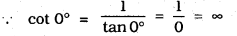∴ When A = 0°, cot A is not defined.

All Chapter KSEEB Solutions For Class 10 Maths

—————————————————————————–

All Subject KSEEB Solutions For Class 10

*************************************************

I think you got complete solutions for this chapter. If You have any queries regarding this chapter, please comment on the below section our subject teacher will answer you. We tried our best to give complete solutions so you got good marks in your exam.

If these solutions have helped you, you can also share kseebsolutionsfor.com to your friends.

Best of Luck!!